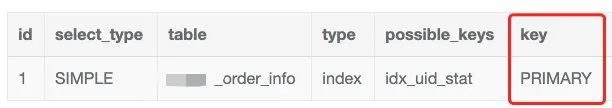# MySQL组合索引，在使用过程中的坑，你遇到了吗？

``select * from order_info where uid = 5837661 order by id asc limit 1``

``CREATE TABLE `order_info` (  `id` bigint(20) unsigned NOT NULL AUTO_INCREMENT,  `uid` int(11) unsigned,  `order_status` tinyint(3) DEFAULT NULL,  ... 省略其它字段和索引  PRIMARY KEY (`id`),  KEY `idx_uid_stat` (`uid`,`order_status`),) ENGINE=InnoDB DEFAULT CHARSET=utf8``

``explain select * from order_info where uid = 5837661 order by id asc limit 1````SET optimizer_trace="enabled=on";        // 打开 optimizer_traceSELECT * FROM order_info where uid = 5837661 order by id asc limit 1SELECT * FROM information_schema.OPTIMIZER_TRACE;    // 查看执行计划表SET optimizer_trace="enabled=off"; // 关闭 optimizer_trace``

MySQL 优化器首先会计算出全表扫描的成本，然后选出该 SQL 可能涉及到的所有索引并且计算索引的成本，然后选出所有成本最小的那个来执行，来看下 optimizer trace 给出的关键信息

``{  "rows_estimation": [    {      "table": "`rebate_order_info`",      "range_analysis": {        "table_scan": {          "rows": 21155996,          "cost": 4.45e6    // 全表扫描成本        }      },      ...      "analyzing_range_alternatives": {          "range_scan_alternatives": [          {            "index": "idx_uid_stat",            "ranges": [            "5837661 <= uid <= 5837661"            ],            "index_dives_for_eq_ranges": true,            "rowid_ordered": false,            "using_mrr": false,            "index_only": false,            "rows": 255918,            "cost": 307103,            // 使用idx_uid_stat索引的成本            "chosen": true            }          ],       "chosen_range_access_summary": {    // 经过上面的各个成本比较后选择的最终结果         "range_access_plan": {             "type": "range_scan",             "index": "idx_uid_stat",  // 可以看到最终选择了idx_uid_stat这个索引来执行             "rows": 255918,             "ranges": [             "58376617 <= uid <= 58376617"             ]         },         "rows_for_plan": 255918,         "cost_for_plan": 307103,         "chosen": true         }         }      ...``

``{    "reconsidering_access_paths_for_index_ordering": {    "clause": "ORDER BY",    "index_order_summary": {      "table": "`rebate_order_info`",      "index_provides_order": true,      "order_direction": "asc",      "index": "PRIMARY",    // 可以看到选择了主键索引      "plan_changed": true,      "access_type": "index_scan"        }    }}``

The short explanation is that the optimizer thinks — or should I say hopes — that scanning the whole table (which is already sorted by the id field) will find the limited rows quick enough, and that this will avoid a sort operation. So by trying to avoid a sort, the optimizer ends-up losing time scanning the table.

1. 使用 force index 来强制使用指定的索引，如下：

``select * from order_info force index(idx_uid_stat) where uid = 5837661 order by id asc limit 1``

1. 使用 order by (id+0) 方案，如下

``select * from order_info where uid = 5837661 order by (id+0) asc limit 1``